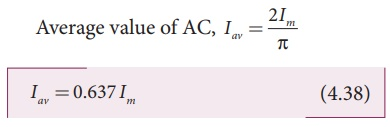Home | | Physics 12th Std | Mean or Average value of AC

# Mean or Average value of AC

The average value of alternating current is defined as the average of all values of current over a positive half-cycle or negative half-cycle.

Mean or Average value of AC

The current and voltage in a DC system remain constant over a period of time so that there is no problem in specifying their magnitudes. However, an alternating current or voltage varies from time to time. Then a question arises how to express the magnitude of an alternating current or voltage. Though there are many ways of expressing it, we limit our discussion with two ways, namely mean value and RMS (Root Mean Square) value of AC.

Mean or Average value of AC

We have learnt that the magnitude of an alternating current in a circuit changes from one instant to other instant and its direction also reverses for every half cycle. During positive half cycle, current is taken as positive and during negative cycle it is negative. Therefore mean or average value of symmetrical alternating current over one complete cycle is zero.

Therefore the average or mean value is measured over one half of a cycle. These electrical terms, average current and average voltage can be used in both AC and DC circuit analysis and calculations.

The average value of alternating current is defined as the average of all values of current over a positive half-cycle or negative half-cycle.

The instantaneous value of sinusoidal alternating current is given by the equation i = I m sin Žē t or i = Im sin╬Ė (where ╬Ė = Žēt) whose graphical representation is given in Figure 4.41.

The sum of all currents over a half-cycle is given by area of positive half-cycle (or negative half-cycle). Therefore,Consider an elementary strip of thickness d╬Ė in the positive half-cycle of the current wave (Figure 4.41). Let i be the mid-ordinate of that strip.Area of the elementary strip = i d╬Ė

Area of positive half-cycleSubstituting  this  in  equation (4.37), we get (The base length of half-cycle is ŽĆ)

Average value of AC, Iav = 2Im/ŽĆHence  the  average  value  of  AC  is 0.637 times the maximum value Im of the alternating current. For negative half-cycle, av = ŌłÆ0.637 Im .

Tags : Alternating Current (AC) , 12th Physics : Electromagnetic Induction and Alternating Current
Study Material, Lecturing Notes, Assignment, Reference, Wiki description explanation, brief detail
12th Physics : Electromagnetic Induction and Alternating Current : Mean or Average value of AC | Alternating Current (AC)